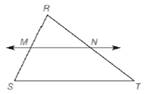Chapter 5.6, Problem 33EElementary Geometry For College St...

7th Edition
Alexander + 2 others
ISBN: 9781337614085

Solutions

Chapter
SectionElementary Geometry For College St...

7th Edition
Alexander + 2 others
ISBN: 9781337614085
Textbook Problem

Use Theorem 5.6.1 and the drawing to complete the proof of this theorem: “If a line is parallel to one side of a triangle and passes through the midpoint of a second side, then it will pass through the midpoint of the third side.” Given: Δ R S T with M the midpoint of R S ; ¯ M N ↔ ∥ S T ¯Prove: N is the midpoint of R T ¯

To determine

To prove:

N is the midpoint of RT¯.

Explanation

Given:

We need to prove, “If a line is parallel to one side of a triangle and passes through the midpoint of a second side, then it will pass through the midpoint of the third side”.

In ΔRST, M is the midpoint of RS¯ and MNST¯.

Property used:

Means-Extremes Property:

In a proportion, the product of the means equals the product of the extremes; that is, if ab=cd (where b0 and d0), then ad=bc.

If a line is parallel to one side of a triangle and intersects other two sides, then it divides these sides proportionally.

Proof:

Consider ΔRST.

Given that M is the midpoint of RS¯.

RM=MS

Given that MNST¯

Still sussing out bartleby?

Check out a sample textbook solution.

See a sample solution

The Solution to Your Study Problems

Bartleby provides explanations to thousands of textbook problems written by our experts, many with advanced degrees!

Get Started

Insert the proper sign to replacein problems 7-14. 10.

Mathematical Applications for the Management, Life, and Social Sciences

Evaluate the expression sin Exercises 116. (14)2

Finite Mathematics and Applied Calculus (MindTap Course List)

If sin=13, find sin3.

Trigonometry (MindTap Course List)

Find f. f(x)=(x+1)/x, f(1) = 5

Single Variable Calculus: Early Transcendentals